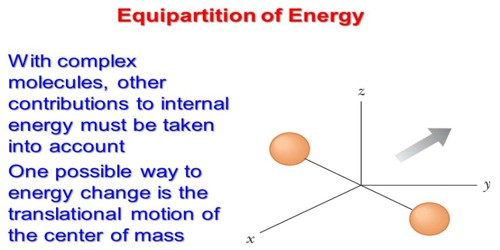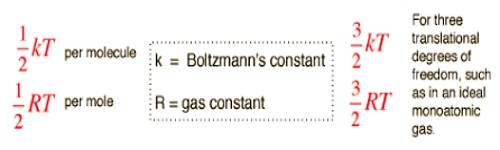# Proof of Law of Equipartition of Energy

Proof of Law of Equipartition of Energy

The law of equipartition of energy states that for any dynamical system in thermal equilibrium, the total energy is equally divided among the degrees of freedom. The law states that: “In thermal equilibrium, the total energy of the molecule is divided equally among all Degrees of Freedom of motion”. The energy associated with a molecule for one degree of freedom is ½ KT where k is the Boltzmann’s constant and T is the temperature.

From kinetic theory of gases we know, in thermal equilibrium average square value of components of velocity of a gas molecule c along three axes X, Y and Z are equally distributed, i.e., u2 = v2 = w2. Here average value of the components of velocities along X, Y and Z axes are respectively u, v and w.

½ mu2 = ½ mv2 = ½ mw2

But c2 = u2 + v2 + w2 and u2 = v2 = w2

so, ½ mu2 = ½ mv2 = ½ mw2 = 1/3 x  ½ mc2

Again, we know, average kinetic energy of each molecule.

½ mc2 = 3/2 KT

Then, ½ mu2 = ½ mv2 = ½ mw2 = ½ x 3/2 KT = ½ KT

So, average energy for each degree of freedom = ½ KT.

Again, in the case of an oscillating particle half of the total energy is kinetic and the rest half is potential energy. So, total energy per degree of freedom = kinetic energy + potential energy = ½ KT + ½ KT = KT.

So, we see that translational kinetic energy associated with each component of velocity is one third at the total energy.The available total energy is equally divided as different independent energy into the components. Before delving into the calculations, let’s understand the law better. If a molecule has 1000 units of energy and 5 degrees of freedom (which includes translational, rotational and vibrational movements), then the molecule allocates 200 units of energy to each motion.

Again molecules are not geometrical points but have infinitely small size. So, molecules have a moment of inertia and mass, hence along with translational motion, there is also rotational motion. Sizes of the molecules are not rigid and due to collision with other molecules, we can expect oscillation in them. As a result their degree of freedom may be more. By Maxwell-Boltzmann’s statistics, it can be shown that energy associated with a degree of freedom becomes the function of an assigned two-dimensional variable of degrees of freedom, then associated average value of energy is equal to ½ KT. If the total energy is distributed equally to all the degrees freedom, then a molecule having degrees of freedom off total energy = f x ½ KT = f/2 KT. Here, instead, is a nonrigorous but plausible derivation based on simple mechanical models. It does not use the distribution function and it shows approximately how long it takes to reach thermal equilibrium.